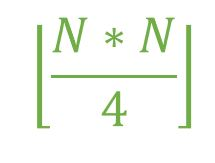GeeksforGeeks App
Open AppBrowser
Continue

# Maximum number of edges in Bipartite graph

Given an integer N which represents the number of Vertices. The Task is to find the maximum number of edges possible in a Bipartite graph of N vertices.
Bipartite Graph:

1. A Bipartite graph is one which is having 2 sets of vertices.
2. The set are such that the vertices in the same set will never share an edge between them.

Examples:

Input: N = 10
Output: 25
Both the sets will contain 5 vertices and every vertex of first set
will have an edge to every other vertex of the second set
i.e. total edges = 5 * 5 = 25
Input: N = 9
Output: 20

Approach: The number of edges will be maximum when every vertex of a given set has an edge to every other vertex of the other set i.e. edges = m * n where m and n are the number of edges in both the sets. in order to maximize the number of edges, m must be equal to or as close to n as possible. Hence, the maximum number of edges can be calculated with the formula,Below is the implementation of the above approach:

## C++

 `// C++ implementation of the approach``#include ``using` `namespace` `std;` `// Function to return the maximum number``// of edges possible in a Bipartite``// graph with N vertices``int` `maxEdges(``int` `N)``{``    ``int` `edges = 0;` `    ``edges = ``floor``((N * N) / 4);` `    ``return` `edges;``}` `// Driver code``int` `main()``{``    ``int` `N = 5;``    ``cout << maxEdges(N);` `    ``return` `0;``}`

## Java

 `// Java implementation of the approach` `class` `GFG {` `    ``// Function to return the maximum number``    ``// of edges possible in a Bipartite``    ``// graph with N vertices``    ``public` `static` `double` `maxEdges(``double` `N)``    ``{``        ``double` `edges = ``0``;` `        ``edges = Math.floor((N * N) / ``4``);` `        ``return` `edges;``    ``}` `    ``// Driver code``    ``public` `static` `void` `main(String[] args)``    ``{``        ``double` `N = ``5``;``        ``System.out.println(maxEdges(N));``    ``}``}` `// This code is contributed by Naman_Garg.`

## Python3

 `# Python3 implementation of the approach` `# Function to return the maximum number``# of edges possible in a Bipartite``# graph with N vertices``def` `maxEdges(N) :` `    ``edges ``=` `0``;` `    ``edges ``=` `(N ``*` `N) ``/``/` `4``;` `    ``return` `edges;` `# Driver code``if` `__name__ ``=``=` `"__main__"` `:``    ` `    ``N ``=` `5``;``    ``print``(maxEdges(N));` `# This code is contributed by AnkitRai01`

## C#

 `// C# implementation of the approach``using` `System;` `class` `GFG {` `    ``// Function to return the maximum number``    ``// of edges possible in a Bipartite``    ``// graph with N vertices``    ``static` `double` `maxEdges(``double` `N)``    ``{``        ``double` `edges = 0;` `        ``edges = Math.Floor((N * N) / 4);` `        ``return` `edges;``    ``}` `    ``// Driver code``    ``static` `public` `void` `Main()``    ``{``        ``double` `N = 5;``        ``Console.WriteLine(maxEdges(N));``    ``}``}` `// This code is contributed by jit_t.`

## PHP

 ``

## Javascript

 ``

Output:

`6`

Time Complexity: O(1)

Auxiliary Space: O(1)

My Personal Notes arrow_drop_up